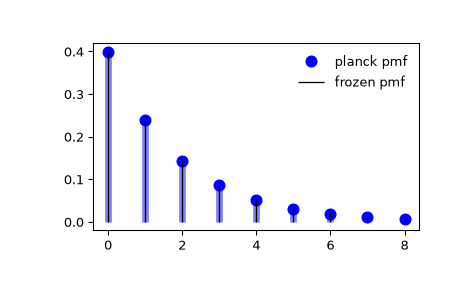# scipy.stats.planck¶

scipy.stats.planck = <scipy.stats._discrete_distns.planck_gen object>[source]

A Planck discrete exponential random variable.

As an instance of the rv_discrete class, planck object inherits from it a collection of generic methods (see below for the full list), and completes them with details specific for this particular distribution.

Notes

The probability mass function for planck is:

$f(k) = (1-\exp(-\lambda)) \exp(-\lambda k)$

for $$k \ge 0$$ and $$\lambda > 0$$.

planck takes $$\lambda$$ as shape parameter. The Planck distribution can be written as a geometric distribution (geom) with $$p = 1 - \exp(-\lambda)$$ shifted by loc = -1.

The probability mass function above is defined in the “standardized” form. To shift distribution use the loc parameter. Specifically, planck.pmf(k, lambda_, loc) is identically equivalent to planck.pmf(k - loc, lambda_).

Examples

>>> from scipy.stats import planck
>>> import matplotlib.pyplot as plt
>>> fig, ax = plt.subplots(1, 1)


Calculate the first four moments:

>>> lambda_ = 0.51
>>> mean, var, skew, kurt = planck.stats(lambda_, moments='mvsk')


Display the probability mass function (pmf):

>>> x = np.arange(planck.ppf(0.01, lambda_),
...               planck.ppf(0.99, lambda_))
>>> ax.plot(x, planck.pmf(x, lambda_), 'bo', ms=8, label='planck pmf')
>>> ax.vlines(x, 0, planck.pmf(x, lambda_), colors='b', lw=5, alpha=0.5)


Alternatively, the distribution object can be called (as a function) to fix the shape and location. This returns a “frozen” RV object holding the given parameters fixed.

Freeze the distribution and display the frozen pmf:

>>> rv = planck(lambda_)
>>> ax.vlines(x, 0, rv.pmf(x), colors='k', linestyles='-', lw=1,
...         label='frozen pmf')
>>> ax.legend(loc='best', frameon=False)
>>> plt.show()Check accuracy of cdf and ppf:

>>> prob = planck.cdf(x, lambda_)
>>> np.allclose(x, planck.ppf(prob, lambda_))
True


Generate random numbers:

>>> r = planck.rvs(lambda_, size=1000)


Methods

 rvs(lambda_, loc=0, size=1, random_state=None) Random variates. pmf(k, lambda_, loc=0) Probability mass function. logpmf(k, lambda_, loc=0) Log of the probability mass function. cdf(k, lambda_, loc=0) Cumulative distribution function. logcdf(k, lambda_, loc=0) Log of the cumulative distribution function. sf(k, lambda_, loc=0) Survival function (also defined as 1 - cdf, but sf is sometimes more accurate). logsf(k, lambda_, loc=0) Log of the survival function. ppf(q, lambda_, loc=0) Percent point function (inverse of cdf — percentiles). isf(q, lambda_, loc=0) Inverse survival function (inverse of sf). stats(lambda_, loc=0, moments=’mv’) Mean(‘m’), variance(‘v’), skew(‘s’), and/or kurtosis(‘k’). entropy(lambda_, loc=0) (Differential) entropy of the RV. expect(func, args=(lambda_,), loc=0, lb=None, ub=None, conditional=False) Expected value of a function (of one argument) with respect to the distribution. median(lambda_, loc=0) Median of the distribution. mean(lambda_, loc=0) Mean of the distribution. var(lambda_, loc=0) Variance of the distribution. std(lambda_, loc=0) Standard deviation of the distribution. interval(alpha, lambda_, loc=0) Endpoints of the range that contains fraction alpha [0, 1] of the distribution